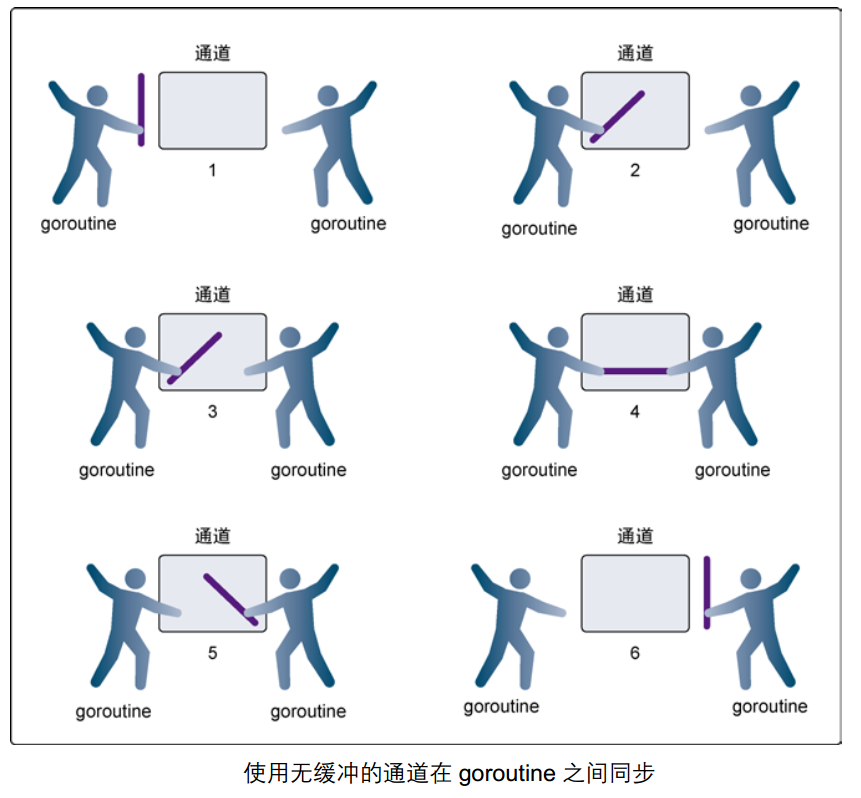# 1. Channel

### 1.1.1. channel

Go语言的并发模型是CSP（Communicating Sequential Processes），提倡通过通信共享内存而不是通过共享内存而实现通信。

Go 语言中的通道（channel）是一种特殊的类型。通道像一个传送带或者队列，总是遵循先入先出（First In First Out）的规则，保证收发数据的顺序。每一个通道都是一个具体类型的导管，也就是声明channel的时候需要为其指定元素类型。

### 1.1.2. channel类型

channel是一种类型，一种引用类型。声明通道类型的格式如下：

``````    var 变量 chan 元素类型
``````

``````    var ch1 chan int   // 声明一个传递整型的通道
var ch2 chan bool  // 声明一个传递布尔型的通道
var ch3 chan []int // 声明一个传递int切片的通道
``````

### 1.1.3. 创建channel

``````var ch chan int
fmt.Println(ch) // <nil>
``````

``````    make(chan 元素类型, [缓冲大小])
``````

channel的缓冲大小是可选的。

``````ch4 := make(chan int)
ch5 := make(chan bool)
ch6 := make(chan []int)
``````

### 1.1.4. channel操作

``````ch := make(chan int)
``````

#### 发送

``````ch <- 10 // 把10发送到ch中
``````

#### 接收

``````x := <- ch // 从ch中接收值并赋值给变量x
<-ch       // 从ch中接收值，忽略结果
``````

#### 关闭

``````    close(ch)
``````

``````    1.对一个关闭的通道再发送值就会导致panic。
2.对一个关闭的通道进行接收会一直获取值直到通道为空。
3.对一个关闭的并且没有值的通道执行接收操作会得到对应类型的零值。
4.关闭一个已经关闭的通道会导致panic。
``````

### 1.1.5. 无缓冲的通道``````func main() {
ch := make(chan int)
ch <- 10
fmt.Println("发送成功")
}
``````

``````    fatal error: all goroutines are asleep - deadlock!

goroutine 1 [chan send]:
main.main()
.../src/github.com/pprof/studygo/day06/channel02/main.go:8 +0x54
``````

``````func recv(c chan int) {
ret := <-c
fmt.Println("接收成功", ret)
}
func main() {
ch := make(chan int)
go recv(ch) // 启用goroutine从通道接收值
ch <- 10
fmt.Println("发送成功")
}
``````

### 1.1.6. 有缓冲的通道``````func main() {
ch := make(chan int, 1) // 创建一个容量为1的有缓冲区通道
ch <- 10
fmt.Println("发送成功")
}
``````

### 1.1.7. close()

``````package main

import "fmt"

func main() {
c := make(chan int)
go func() {
for i := 0; i < 5; i++ {
c <- i
}
close(c)
}()
for {
if data, ok := <-c; ok {
fmt.Println(data)
} else {
break
}
}
fmt.Println("main结束")
}
``````

### 1.1.8. 如何优雅的从通道循环取值

``````// channel 练习
func main() {
ch1 := make(chan int)
ch2 := make(chan int)
// 开启goroutine将0~100的数发送到ch1中
go func() {
for i := 0; i < 100; i++ {
ch1 <- i
}
close(ch1)
}()
// 开启goroutine从ch1中接收值，并将该值的平方发送到ch2中
go func() {
for {
i, ok := <-ch1 // 通道关闭后再取值ok=false
if !ok {
break
}
ch2 <- i * i
}
close(ch2)
}()
// 在主goroutine中从ch2中接收值打印
for i := range ch2 { // 通道关闭后会退出for range循环
fmt.Println(i)
}
}
``````

### 1.1.9. 单向通道

Go语言中提供了单向通道来处理这种情况。例如，我们把上面的例子改造如下：

``````func counter(out chan<- int) {
for i := 0; i < 100; i++ {
out <- i
}
close(out)
}

func squarer(out chan<- int, in <-chan int) {
for i := range in {
out <- i * i
}
close(out)
}
func printer(in <-chan int) {
for i := range in {
fmt.Println(i)
}
}

func main() {
ch1 := make(chan int)
ch2 := make(chan int)
go counter(ch1)
go squarer(ch2, ch1)
printer(ch2)
}
``````

``````    1.chan<- int是一个只能发送的通道，可以发送但是不能接收；
2.<-chan int是一个只能接收的通道，可以接收但是不能发送。
``````

### 1.1.10. 通道总结

channel常见的异常总结，如下图：